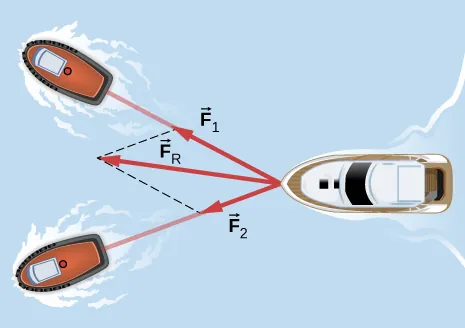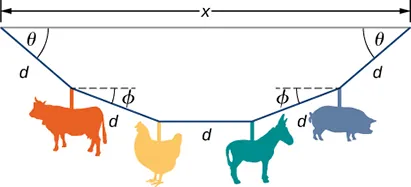University Physics Volume 1

# Challenge Problems

University Physics Volume 1Challenge Problems

### Challenge Problems

93.

If two tugboats pull on a disabled vessel, as shown here in an overhead view, the disabled vessel will be pulled along the direction indicated by the result of the exerted forces. (a) Draw a free-body diagram for the vessel. Assume no friction or drag forces affect the vessel. (b) Did you include all forces in the overhead view in your free-body diagram? Why or why not?94.

A 10.0-kg object is initially moving east at 15.0 m/s. Then a force acts on it for 2.00 s, after which it moves northwest, also at 15.0 m/s. What are the magnitude and direction of the average force that acted on the object over the 2.00-s interval?

95.

On June 25, 1983, shot-putter Udo Beyer of East Germany threw the 7.26-kg shot 22.22 m, which at that time was a world record. (a) If the shot was released at a height of 2.20 m with a projection angle of $45.0°45.0°$, what was its initial velocity? (b) If while in Beyer’s hand the shot was accelerated uniformly over a distance of 1.20 m, what was the net force on it?

96.

A body of mass m moves in a horizontal direction such that at time t its position is given by $x(t)=at4+bt3+ct,x(t)=at4+bt3+ct,$ where a, b, and c are constants. (a) What is the acceleration of the body? (b) What is the time-dependent force acting on the body?

97.

A body of mass m has initial velocity $v0v0$ in the positive x-direction. It is acted on by a constant force F for time t until the velocity becomes zero; the force continues to act on the body until its velocity becomes $−v0−v0$ in the same amount of time. Write an expression for the total distance the body travels in terms of the variables indicated.

98.

The velocities of a 3.0-kg object at $t=6.0st=6.0s$ and $t=8.0st=8.0s$ are $(3.0i^−6.0j^+4.0k^)m/s(3.0i^−6.0j^+4.0k^)m/s$ and $(−2.0i^+4.0k^)m/s(−2.0i^+4.0k^)m/s$, respectively. If the object is moving at constant acceleration, what is the force acting on it?

99.

A 120-kg astronaut is riding in a rocket sled that is sliding along an inclined plane. The sled has a horizontal component of acceleration of $5.0m/s25.0m/s2$ and a downward component of $3.8m/s23.8m/s2$. Calculate the magnitude of the force on the rider by the sled. (Hint: Remember that gravitational acceleration must be considered.)

100.

Two forces are acting on a 5.0-kg object that moves with acceleration $2.0m/s22.0m/s2$ in the positive y-direction. If one of the forces acts in the positive x-direction and has magnitude of 12 N, what is the magnitude of the other force?

101.

Suppose that you are viewing a soccer game from a helicopter above the playing field. Two soccer players simultaneously kick a stationary soccer ball on the flat field; the soccer ball has mass 0.420 kg. The first player kicks with force 162 N at $9.0°9.0°$ north of west. At the same instant, the second player kicks with force 215 N at $15°15°$ east of south. Find the acceleration of the ball in $i^i^$ and $j^j^$ form.

102.

A 10.0-kg mass hangs from a spring that has the spring constant 535 N/m. Find the position of the end of the spring away from its rest position. (Use $g=9.80m/s2g=9.80m/s2$.)

103.

A 0.0502-kg pair of fuzzy dice is attached to the rearview mirror of a car by a short string. The car accelerates at constant rate, and the dice hang at an angle of $3.20°3.20°$ from the vertical because of the car’s acceleration. What is the magnitude of the acceleration of the car?

104.

At a circus, a donkey pulls on a sled carrying a small clown with a force given by $2.48i^+4.33j^N2.48i^+4.33j^N$. A horse pulls on the same sled, aiding the hapless donkey, with a force of $6.56i^+5.33j^N6.56i^+5.33j^N$. The mass of the sled is 575 kg. Using $i^i^$ and $j^j^$ form for the answer to each problem, find (a) the net force on the sled when the two animals act together, (b) the acceleration of the sled, and (c) the velocity after 6.50 s.

105.

Hanging from the ceiling over a baby bed, well out of baby’s reach, is a string with plastic shapes, as shown here. The string is taut (there is no slack), as shown by the straight segments. Each plastic shape has the same mass m, and they are equally spaced by a distance d, as shown. The angles labeled $θθ$ describe the angle formed by the end of the string and the ceiling at each end. The center length of sting is horizontal. The remaining two segments each form an angle with the horizontal, labeled $ϕϕ$. Let $T1T1$ be the tension in the leftmost section of the string, $T2T2$ be the tension in the section adjacent to it, and $T3T3$ be the tension in the horizontal segment. (a) Find an equation for the tension in each section of the string in terms of the variables m, g, and $θθ$. (b) Find the angle $ϕϕ$ in terms of the angle $θθ$. (c) If $θ=5.10°θ=5.10°$, what is the value of $ϕϕ$? (d) Find the distance x between the endpoints in terms of d and $θθ$.106.

A bullet shot from a rifle has mass of 10.0 g and travels to the right at 350 m/s. It strikes a target, a large bag of sand, penetrating it a distance of 34.0 cm. Find the magnitude and direction of the retarding force that slows and stops the bullet.

107.

An object is acted on by three simultaneous forces: $F→1=(−3.00i^+2.00j^)NF→1=(−3.00i^+2.00j^)N$, $F→2=(6.00i^−4.00j^)NF→2=(6.00i^−4.00j^)N$, and $F→3=(2.00i^+5.00j^)NF→3=(2.00i^+5.00j^)N$. The object experiences acceleration of $4.23m/s24.23m/s2$. (a) Find the acceleration vector in terms of m. (b) Find the mass of the object. (c) If the object begins from rest, find its speed after 5.00 s. (d) Find the components of the velocity of the object after 5.00 s.

108.

In a particle accelerator, a proton has mass $1.67×10−27kg1.67×10−27kg$ and an initial speed of $2.00×105m/s.2.00×105m/s.$ It moves in a straight line, and its speed increases to $9.00×105m/s9.00×105m/s$ in a distance of 10.0 cm. Assume that the acceleration is constant. Find the magnitude of the force exerted on the proton.

109.

A drone is being directed across a frictionless ice-covered lake. The mass of the drone is 1.50 kg, and its velocity is $3.00i^m/s3.00i^m/s$. After 10.0 s, the velocity is $9.00i^+4.00j^m/s9.00i^+4.00j^m/s$. If a constant force in the horizontal direction is causing this change in motion, find (a) the components of the force and (b) the magnitude of the force.

Order a print copy

As an Amazon Associate we earn from qualifying purchases.